Courses

# Displacement Methods: Slope Deflection and Moment Distribution Methods Notes | EduRev

## GATE : Displacement Methods: Slope Deflection and Moment Distribution Methods Notes | EduRev

The document Displacement Methods: Slope Deflection and Moment Distribution Methods Notes | EduRev is a part of the GATE Course Structural Analysis.
All you need of GATE at this link: GATE

Strain Energy Method

1. Strain energy stored due to axial load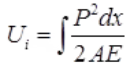dx = Elemental length
AE = Axial rigidity
2. Strain energy stored due to bending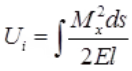where, MX = Bending moment at section x-x
ds = Elemental length
El = Flexural rigidity
or E = Modulus of elasticity
l = Moment of inertia
3. Strain energy stored due to shear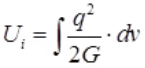where, q = Shear stress
G = Modulus of rigidity
dv = Elemental volume
4. Strain energy stored due to shear force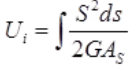where, AS = Area of shear
S = Shear force
G = Modulus of rigidity
ds = Elemental length
5. Strain energy stored due to torsion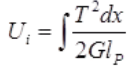where, T = Torque acting on circular bar
dx = Elemental length
G = Modulus of rigidity
lP = Polar moment of inertia
6. Strain energy stored in terms of maximum shear stress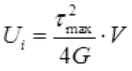where, image007 Maximum shear stress at the surface of rod under twisting.
G = Modulus of rigidity
V = Volume
7. Strain energy stored in hollow circular shaft is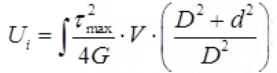Where, D = External dia of hollow circular shafts
d = Internal dia of hollow circular shaft
τmax = Maximum shear stress

Castigliano's first theorem

∂U/∂Δ = P & ∂U/∂θ = M
where, U = Total strain energy
Δ = Displacement in the direction of force P.
θ = Rotation in the direction of moment M.

Castiglianos Second Theorem

∂U/∂P = Δ, ∂U/∂M = θ

Betti's Law

∑Pmδmn = ∑Pnδnm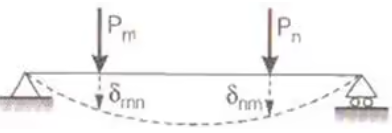where, Pm = Load applied in the direction m.
Pn = Load applied in the direction n.
δmn = Deflection in the direction 'm' due to load applied in the direction 'n'.
δnm = Deflection in the direction 'n' due to load applied in the direction 'm'.

Maxwells Reciprocal Theorem

δ21 = δ12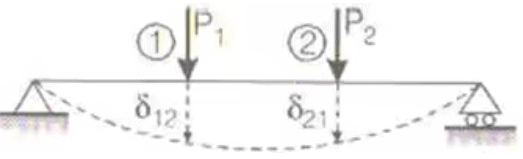where, δ21 = deflection in the direction (2) due to applied load in the direction (1).
δ12 = Deflection in the direction (1) due to applied load in the direction (2).

Standard Cases of Deflection

1. For the portal frame shown in the figure below horizontal deflection at D due to load P, assuming all members have same flexural rigidity is given by
δ = Ph2(2h + 3b)/3El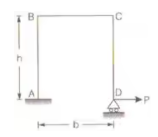where, δ = Deflection of D in the direction of load P.
2. Semicircular arch whose one end is hinged and other supported on roller carried a load P as shown in the figure.
δ = π/2.PR3/El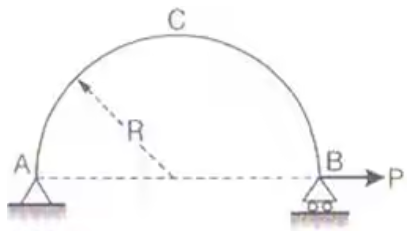where, δ = Deflection at B in the direction of load P.
3. A quadrantal ring AB of radius r support a concentrated load P as shown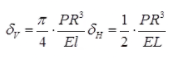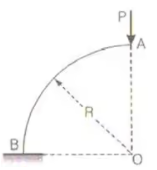where, δV = Vertical deflection (deflection in the direction of load P) at end A
δH = Horizontal deflection of end A.
4. A portal frame as shown in figure carries a load P at A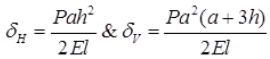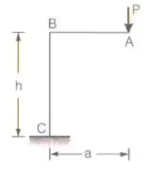where, δH & δV is horizontal & vertical deflection at end A respectively.
5. Figure shows two identical wires OA and OB each of area A and inclined at 45° from horizontal. A load P is supported at O
δv = PL/AE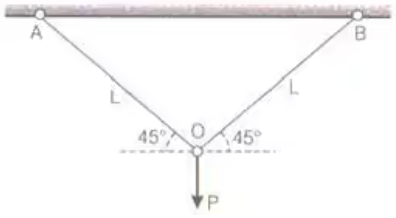where, δV = Vertical deflection at 'O'.

Moment distribution method (Hardy Cross method)

1. Stiffness: It is the force/moment required to be applied at a joint so as to produce unit deflection/rotation at that joint.
k = F/Δ or M/θ
Where, K = Stiffness
F = Force required to produce deflection Δ
M = Moment required to produce rotation θ.
2. Stiffness of beam
(i) Stiffness of member BA when farther end A is fixed.
k = 4El/L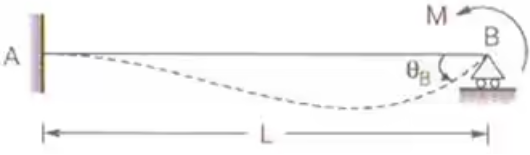Where, K = Stiffness of BA at joint B. When farther end is fixed.
El = Flexural rigidity
L = Length of the beam
M = Moment at B.
(ii) Stiffness of member BA when farther end A is hinged
k = 4El/L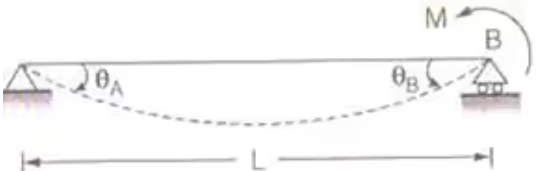where, K = Stiffness of BA at joint B. When farther end is hinged
3. Carry over factor
Carry over factor = Carry over moment/Applied moment
COF may greater than, equal to or less than 1.
4. Standard Cases
(i) COF = 1/2
(ii) COF = 0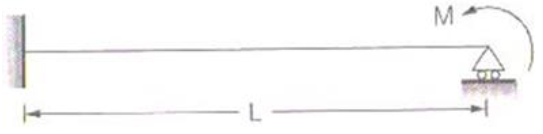(iii) COF = a/b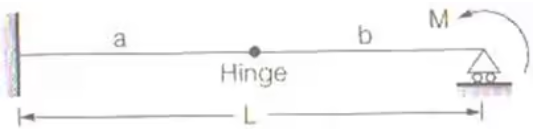5. Distribution Factor (D.F.)
DF = stiffness of a member/Sum of stiffness of all members at that joint
or
DF= Relative stiffness of a member/Sum of relative stiffness of all member at that joint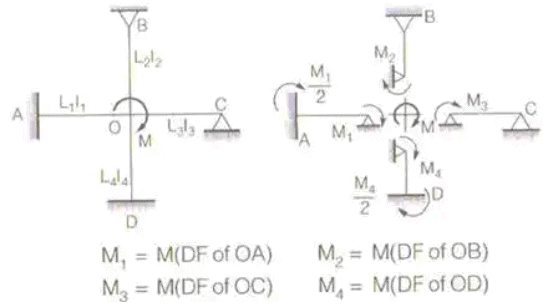where M1, M2, M3 and M4 are moment induced in member OA, OB, OC and OD respectively.
6. Relative Stiffness
(i) When farther end is fixed
Relative stiffness for member = l/L
(ii) When farther end is hinged
Relative stiffness for member = 3l/4L
Where, l = MOl and L = Length of Beam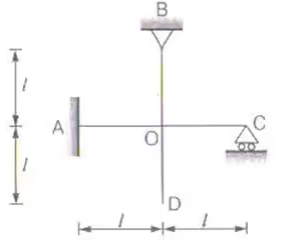Stiffness of OA = 4El/l
Stiffness of OB
Stiffness of OC = 34El/l
Stiffness of OD = 0
7. Fixed Convention
+ve → Sagging
–ve → Hogging
and All clockwise moment → +ve
and All Anti clockwise moment → –ve
Span length is l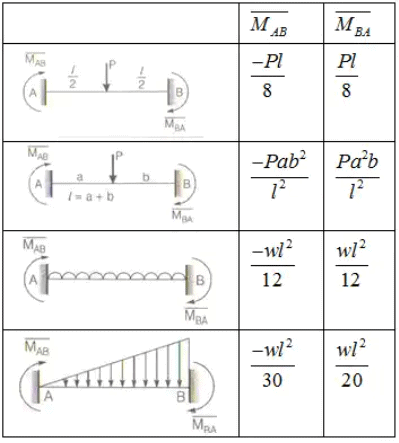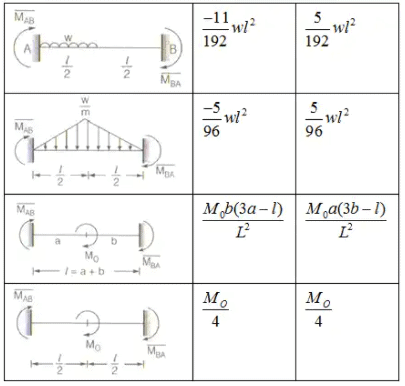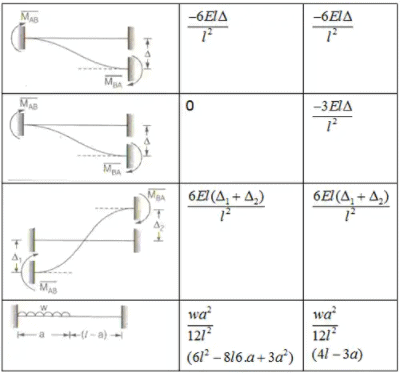Slope deflection Method (G.A. Maney Method)

In this method, joints are considered rigid. It means joints rotate as a whole and the angles between the tangents to the elastic curve meeting at that joint do not change due to rotation. The basic unknown are joint displacement (θ and Δ).
To find θ and Δ, joints equilibrium conditions and shear equations are established. The forces (moments) are found using force displacement relations. Which are called slope deflection equations.

Slope Deflection Equation
(i) The slope deflection equation at the end A for member AB can be written as: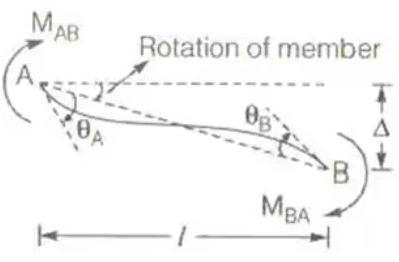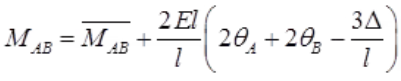(ii) The slope deflection equation at the end B for member BA can be written as: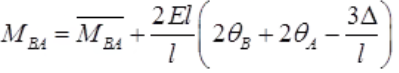where, L = Length of beam, El = Flexural Rigidity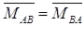are fixed end moments at A & B respectively. MAB & MBA are final moments at A & B respectively. θA and θB are rotation of joint A & B respectively.
Δ = Settlement of support
Sign Convention
+M → Clockwise
-M → Anti-clockwise
+θ → Clockwise
-θ → Anti-clockwise
Δ → +ve, if it produces clockwise rotation to the member & vice-versa.
The number of joint equilibrium conditions will be equal to number of ‘θ’ components & number of shear equations will be equal to number of ‘Δ’ Components.

Offer running on EduRev: Apply code STAYHOME200 to get INR 200 off on our premium plan EduRev Infinity!

## Structural Analysis

30 videos|122 docs|28 tests

,

,

,

,

,

,

,

,

,

,

,

,

,

,

,

,

,

,

,

,

,

;